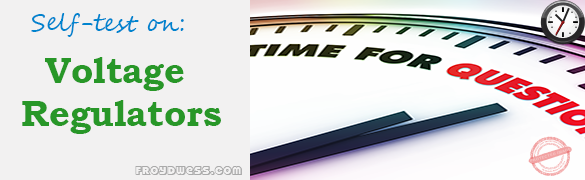# Floyd Self-test in Voltage Regulators

(Last Updated On: December 8, 2017)This is the Self-test in Chapter 17: Voltage Regulators from the book Electronic Devices Conventional Current Version, 9th edition by Thomas L. Floyd. If you are looking for a reviewer in Electronics Engineering this will definitely help you before taking the Board Exam.

### Floyd Self-test Chapter 17 Topic Outline

• Floyd Self-test in Voltage Regulation
• Floyd Self-test in Basic Linear Series Regulators
• Floyd Self-test in Basic Linear Shunt Regulators
• Floyd Self-test in Basic Switching Regulators
• Floyd Self-test in Integrated Circuit Voltage Regulators
• Floyd Self-test in Integrated Circuit Voltage Regulator Configurations

### Start Practice Exam Test Questions

Choose the letter of the best answer in each questions.

1. In the case of line regulation,

• (a) when the temperature varies, the output voltage stays constant
• (b) when the output voltage changes, the load current stays constant
• (c) when the input voltage changes, the output voltage stays constant
• (d) when the load changes, the output voltage stays constant

2. In the case of load regulation,

• (a) when the temperature varies, the output voltage stays constant
• (b) when the input voltage changes, the load current stays constant
• (c) when the load changes, the load current stays constant
• (d) when the load changes, the output voltage stays constant

3. All of the following are parts of a basic voltage regulator except

• (a) control element
• (b) sampling circuit
• (c) voltage-follower
• (d) error detector
• (e) reference voltage

4. The basic difference between a series regulator and a shunt regulator is

• (a) the amount of current that can be handled
• (b) the position of the control element
• (c) the type of sample circuit
• (d) the type of error detector

5. In a basic series regulator, is determined by

• (a) the control element
• (b) the sample circuit
• (c) the reference voltage
• (d) answers (b) and (c)

6. The main purpose of current limiting in a regulator is

• (a) protection of the regulator from excessive current
• (b) protection of the load from excessive current
• (c) to keep the power supply transformer from burning up
• (d) to maintain a constant output voltage

7. In a linear regulator, the control transistor is conducting

• (a) a small part of the time
• (b) half the time
• (c) all of the time
• (d) only when the load current is excessive

8. In a basic shunt regulator, is determined by

• (a) the control element
• (b) the sample circuit
• (c) the reference voltage
• (d) answers (b) and (c)

9. In a switching regulator, the control transistor is conducting

• (a) part of the time
• (b) all of the time
• (c) only when the input voltage exceeds a set limit
• (d) only when there is an overload

10. The LM317 is an example of an IC

• (a) three-terminal negative voltage regulator (b) fixed positive voltage regulator
• (c) switching regulator
• (d) linear regulator
• (e) variable positive voltage regulator
• (f ) answers (b) and (d) only
• (g) answers (d) and (e) only

11. An external pass transistor is used for

• (a) increasing the output voltage
• (b) improving the regulation
• (c) increasing the current that the regulator can handle
• (d) short-circuit protection

Rate this: